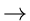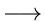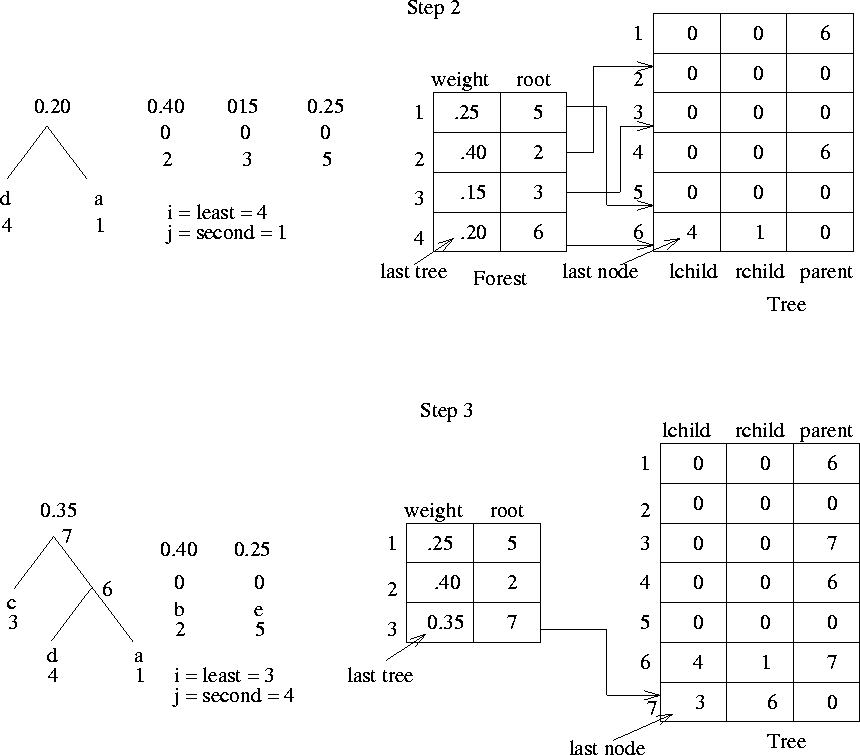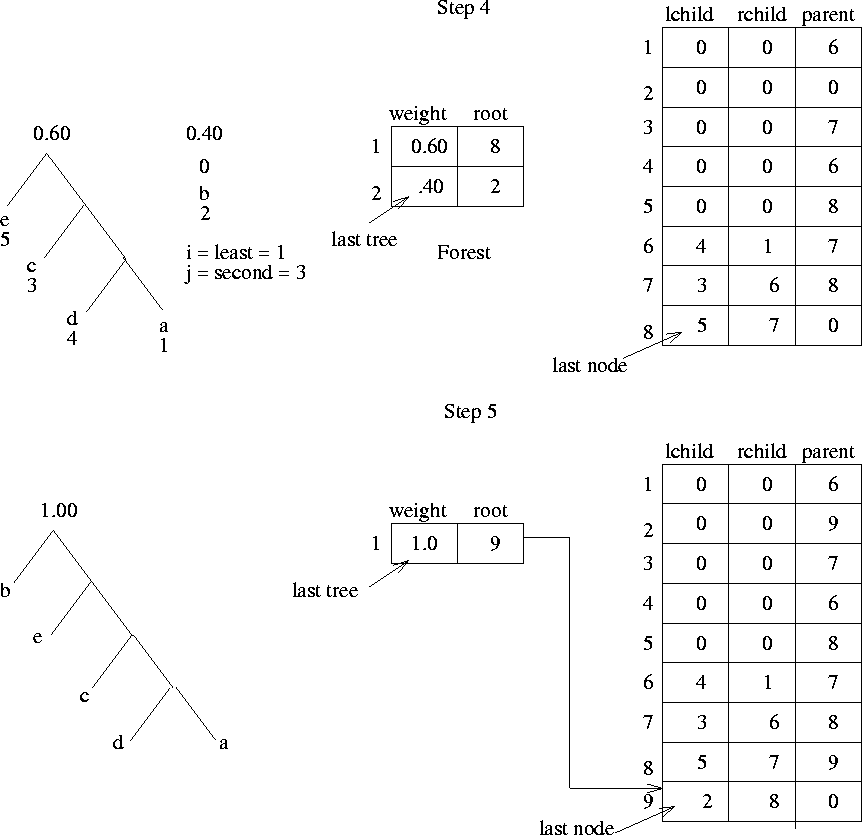Next: 4.4 Binary Search Tree Up: 4.3 An Application of Binary Trees: Huffman Code Construction Previous: 4.3.1 Implementation

## 4.3.2 Sketch of Huffman Tree Construction

1.
while (there is more than one tree in the forest) do {
2.
i = index of tree in forest with smallest weight ;
3.
j = index of tree in forest with second smallest weight ;
4.
create a new node with
lchild = forest [i].root ;
rchild = forest [j].root ;
5.
replace tree i in forest by a tree with root as new node, with
weight = forest [i]weight + forest[j]weight ;
6.
delete tree j from forest
}
Line (4)increases the number of cells of the array tree. Lines (5) and (6)decrease the number of utilized cells of forest. Figures 4.11, 4.12, and 4.13 illustrate different steps of the above algorithm for our example problem.Next: 4.4 Binary Search Tree Up: 4.3 An Application of Binary Trees: Huffman Code Construction Previous: 4.3.1 Implementation
eEL,CSA_Dept,IISc,Bangalore